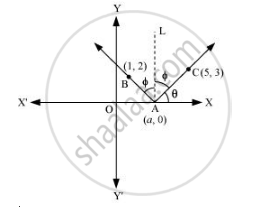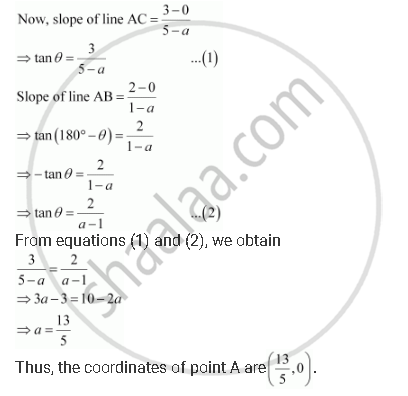CBSE (Arts) Class 11CBSE
Share

# A Ray of Light Passing Through the Point (1, 2) Reflects on the X-axis at Point a and the Reflected Ray Passes Through the Point (5, 3). Find the Coordinates of A. - CBSE (Arts) Class 11 - Mathematics

ConceptDistance of a Point from a Line

#### Question

A ray of light passing through the point (1, 2) reflects on the x-axis at point A and the reflected ray passes through the point (5, 3). Find the coordinates of A.

#### SolutionLet the coordinates of point A be (a, 0).

Draw a line (AL) perpendicular to the x-axis.

We know that angle of incidence is equal to angle of reflection. Hence, let

∠BAL = ∠CAL = Φ

Let ∠CAX = θ

∴∠OAB = 180° – (θ + 2Φ) = 180° – [θ + 2(90° – θ)]

= 180° – θ – 180° + 2θ

θ

∴∠BAX = 180° – θIs there an error in this question or solution?

#### Video TutorialsVIEW ALL 

Solution A Ray of Light Passing Through the Point (1, 2) Reflects on the X-axis at Point a and the Reflected Ray Passes Through the Point (5, 3). Find the Coordinates of A. Concept: Distance of a Point from a Line.
S